# Java Script 1 Java Script Basics Introduction n

• Slides: 53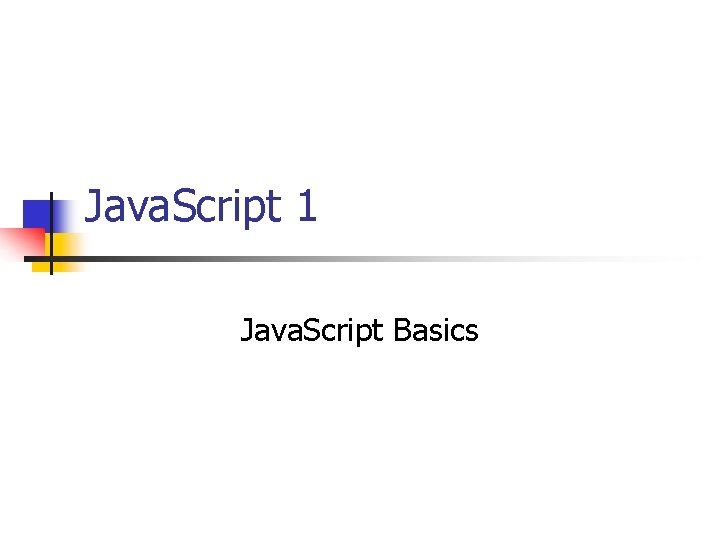Java. Script 1 Java. Script Basics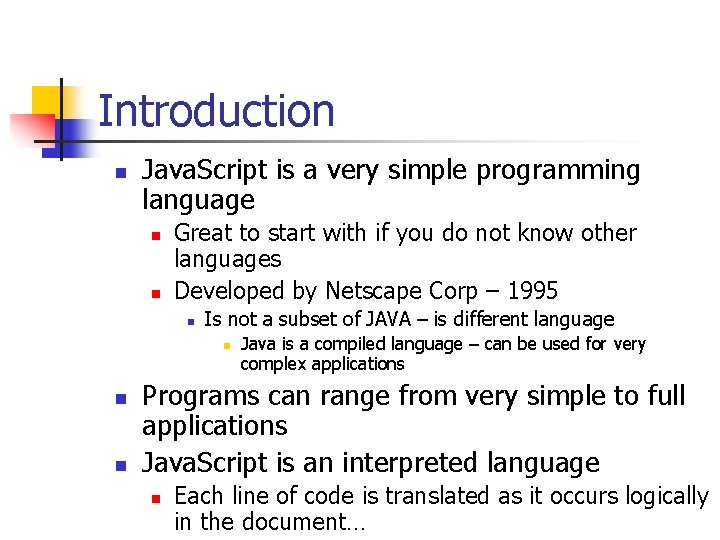Introduction n Java. Script is a very simple programming language n n Great to start with if you do not know other languages Developed by Netscape Corp – 1995 n Is not a subset of JAVA – is different language n n n Java is a compiled language – can be used for very complex applications Programs can range from very simple to full applications Java. Script is an interpreted language n Each line of code is translated as it occurs logically in the document…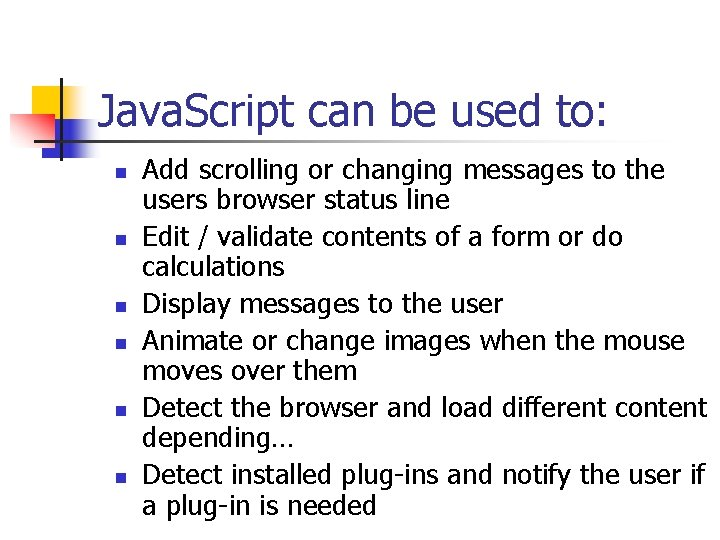Java. Script can be used to: n n n Add scrolling or changing messages to the users browser status line Edit / validate contents of a form or do calculations Display messages to the user Animate or change images when the mouse moves over them Detect the browser and load different content depending… Detect installed plug-ins and notify the user if a plug-in is needed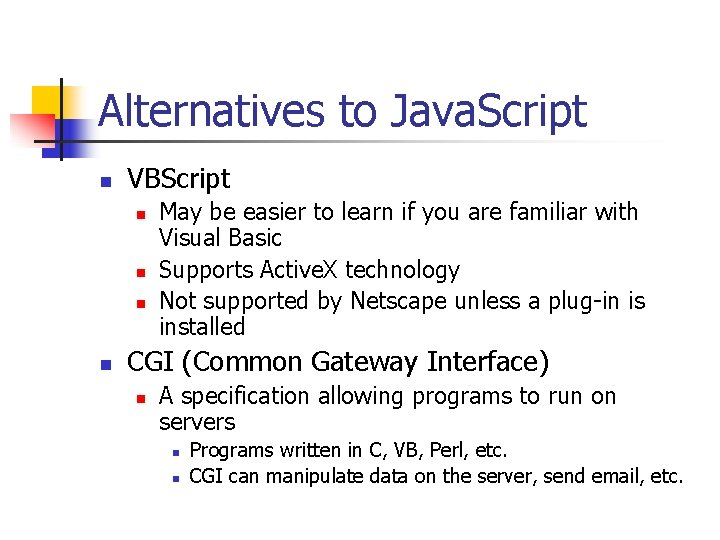Alternatives to Java. Script n VBScript n n May be easier to learn if you are familiar with Visual Basic Supports Active. X technology Not supported by Netscape unless a plug-in is installed CGI (Common Gateway Interface) n A specification allowing programs to run on servers n n Programs written in C, VB, Perl, etc. CGI can manipulate data on the server, send email, etc.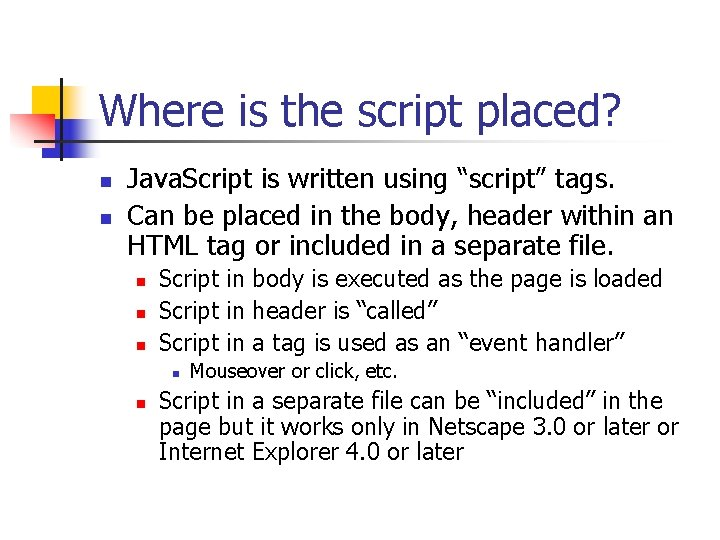Where is the script placed? n n Java. Script is written using “script” tags. Can be placed in the body, header within an HTML tag or included in a separate file. n n n Script in body is executed as the page is loaded Script in header is “called” Script in a tag is used as an “event handler” n n Mouseover or click, etc. Script in a separate file can be “included” in the page but it works only in Netscape 3. 0 or later or Internet Explorer 4. 0 or later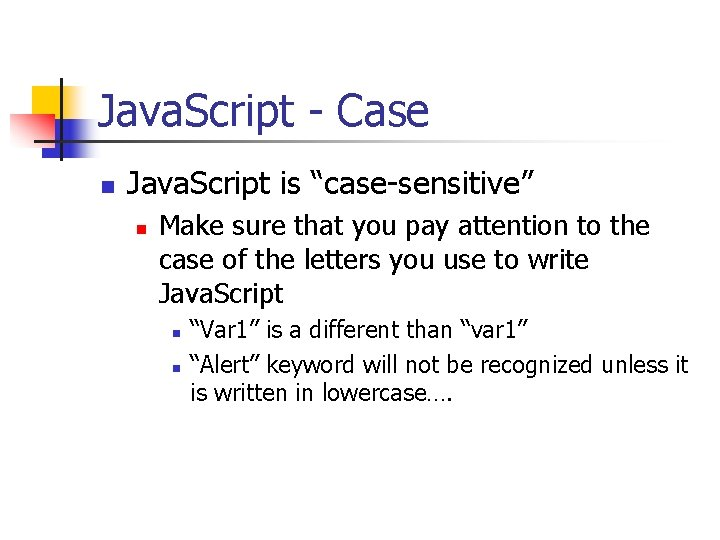Java. Script - Case n Java. Script is “case-sensitive” n Make sure that you pay attention to the case of the letters you use to write Java. Script n n “Var 1” is a different than “var 1” “Alert” keyword will not be recognized unless it is written in lowercase….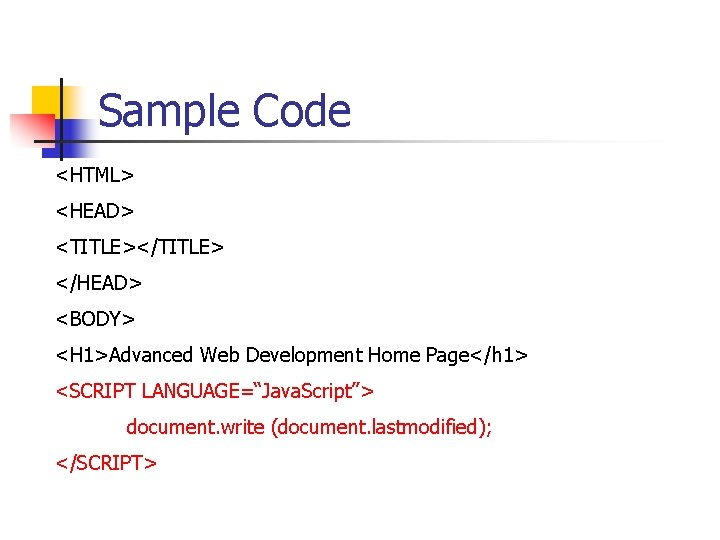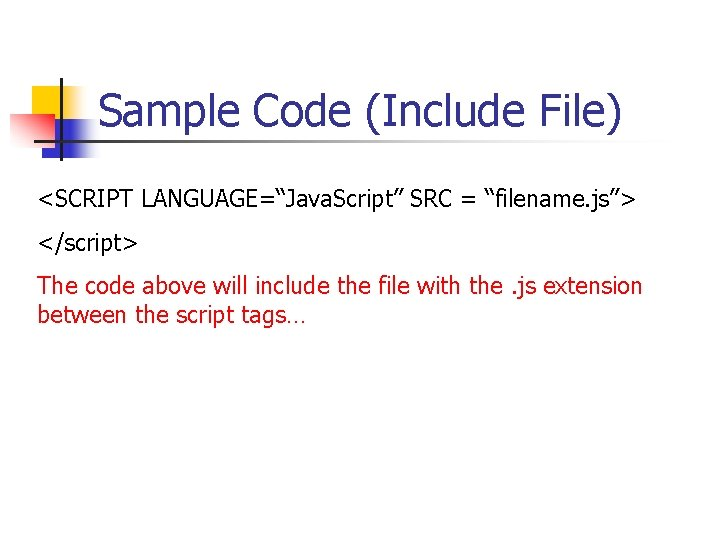Sample Code (Include File) <SCRIPT LANGUAGE=“Java. Script” SRC = “filename. js”> </script> The code above will include the file with the. js extension between the script tags…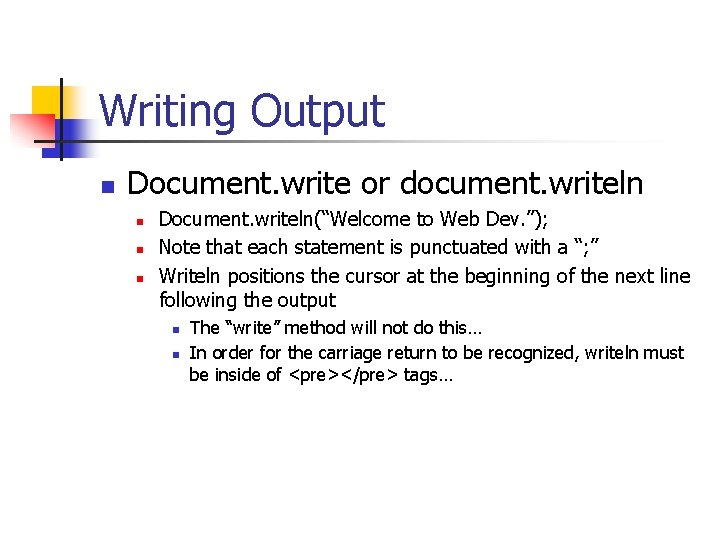Writing Output n Document. write or document. writeln n Document. writeln(“Welcome to Web Dev. ”); Note that each statement is punctuated with a “; ” Writeln positions the cursor at the beginning of the next line following the output n n The “write” method will not do this… In order for the carriage return to be recognized, writeln must be inside of <pre></pre> tags…Sending messages n The Alert method of the window object is used to send a message to the user in the form of a “message box” n n n window. alert (“Hello World”); Use of the “n” newline escape character will allow multiple lines of output in the box: window. alert(“Hellon How are you? ”);Input – window. prompt n User input can be accomplished via the window object’s “prompt” method n n n Brings up a separate window with a textbox, OK and Cancel buttons Var 1 = window. prompt(“Enter a number”, 0) Where the first parameter is the string to prompt the user and the second is the default value of the input box…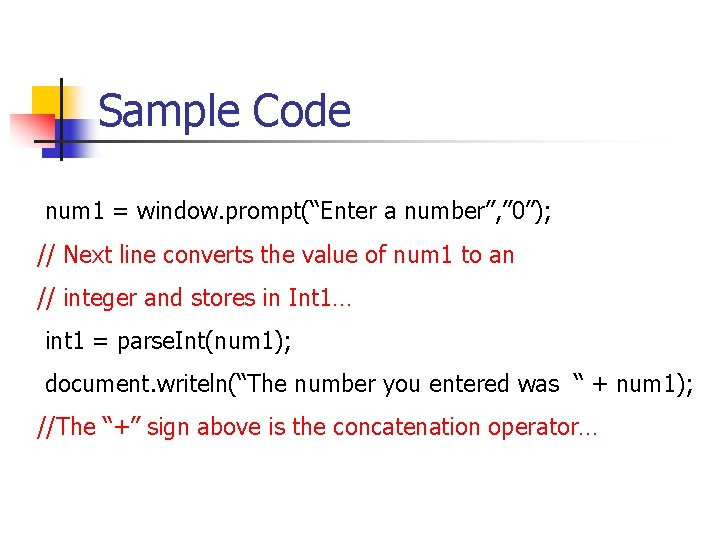Sample Code num 1 = window. prompt(“Enter a number”, ” 0”); // Next line converts the value of num 1 to an // integer and stores in Int 1… int 1 = parse. Int(num 1); document. writeln(“The number you entered was “ + num 1); //The “+” sign above is the concatenation operator…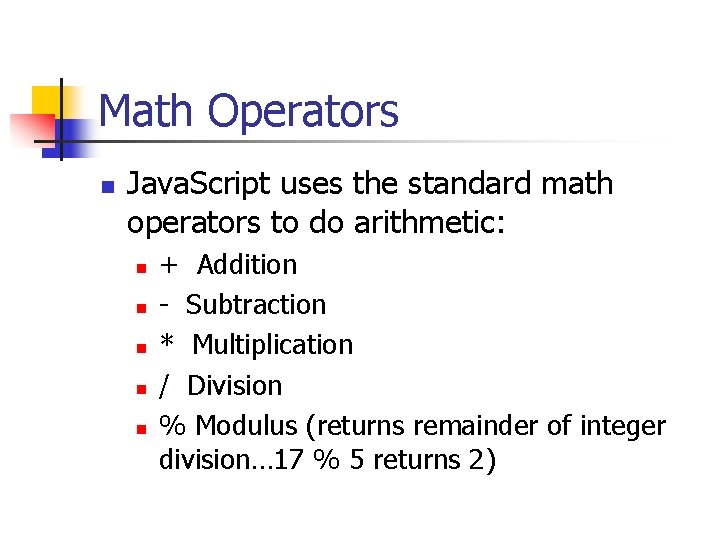Math Operators n Java. Script uses the standard math operators to do arithmetic: n n n + Addition - Subtraction * Multiplication / Division % Modulus (returns remainder of integer division… 17 % 5 returns 2)Hierarchy of Math Operations n () Parenthesis are evaluated first from inner to outer or from left to right if used at the same level n n n ((n + a) – 6) or (n + a) – (6 + 0) * or / or % Evaluated from left to right + or – Evaluated last from left to right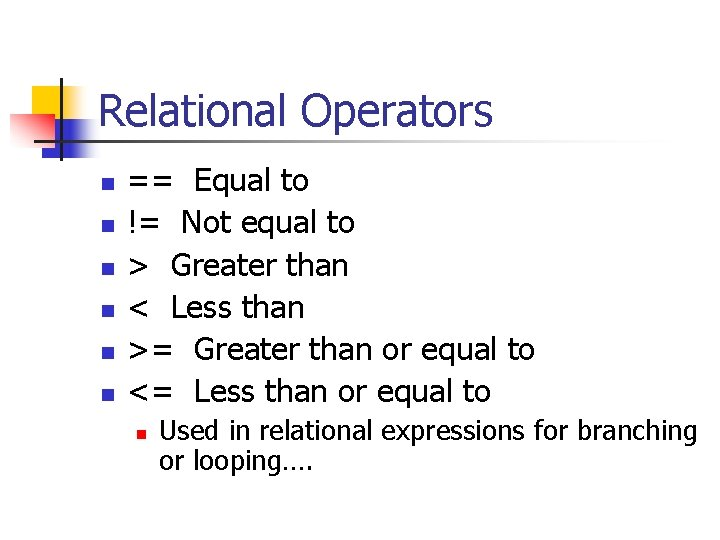Relational Operators n n n == Equal to != Not equal to > Greater than < Less than >= Greater than or equal to <= Less than or equal to n Used in relational expressions for branching or looping….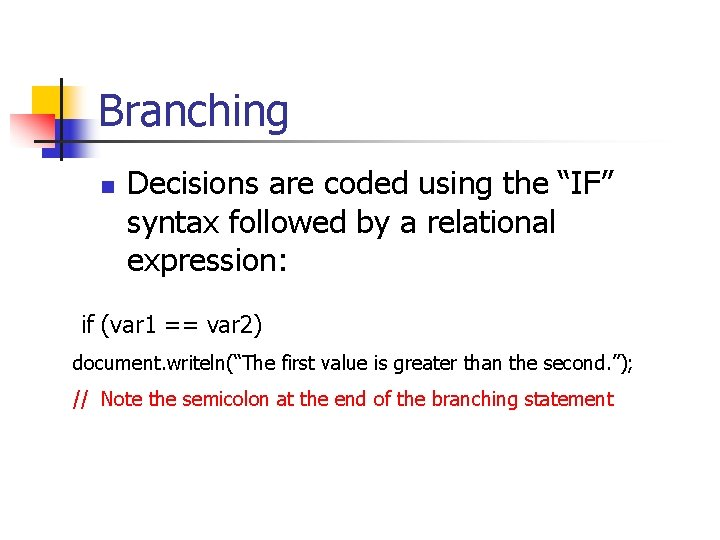Branching n Decisions are coded using the “IF” syntax followed by a relational expression: if (var 1 == var 2) document. writeln(“The first value is greater than the second. ”); // Note the semicolon at the end of the branching statement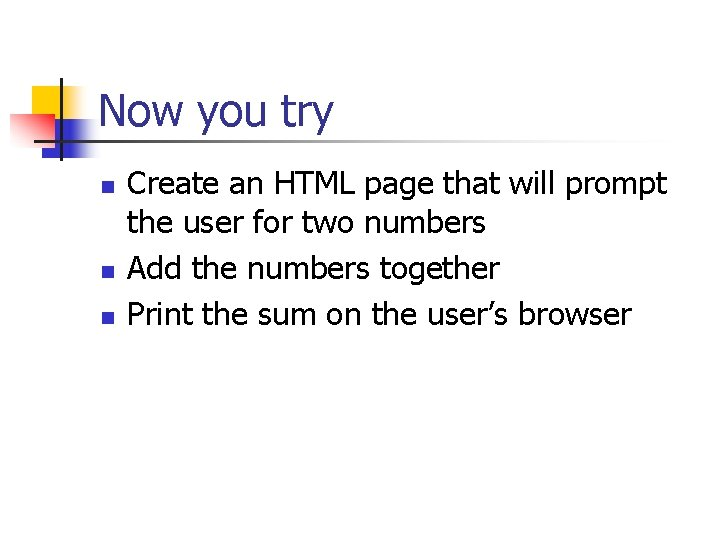Now you try n n n Create an HTML page that will prompt the user for two numbers Add the numbers together Print the sum on the user’s browserNow you try n n n Write a script that allows the user to enter a number Determine whether the number is odd or even Print a message to the user using the “alert” method indicating the result…How Java. Script works n In the following example note that the code is called twice. n The code in the Head section is written in a Function n Is executed when the function is called twice from within the Body of the page. n n n Call a function using its name Note the braces around the function code Note the argument in the function heading “who” n The value for this is passed when the function is called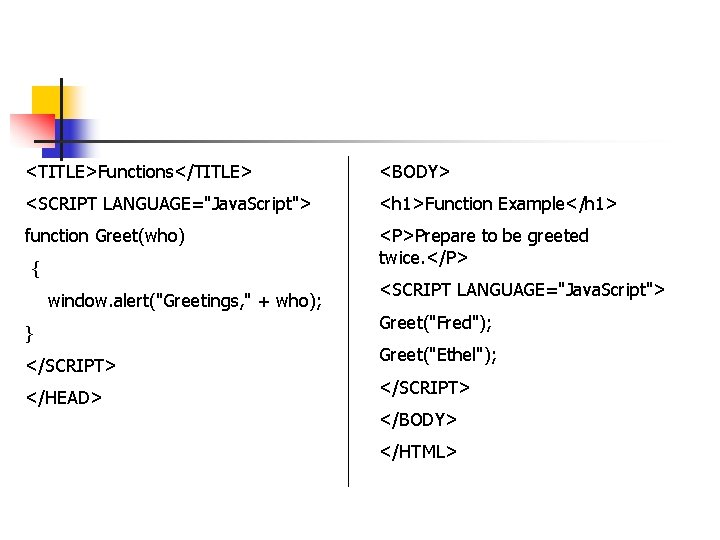<TITLE>Functions</TITLE> <BODY> <SCRIPT LANGUAGE="Java. Script"> <h 1>Function Example</h 1> function Greet(who) <P>Prepare to be greeted twice. </P> { window. alert("Greetings, " + who); } </SCRIPT> </HEAD> <SCRIPT LANGUAGE="Java. Script"> Greet("Fred"); Greet("Ethel"); </SCRIPT> </BODY> </HTML>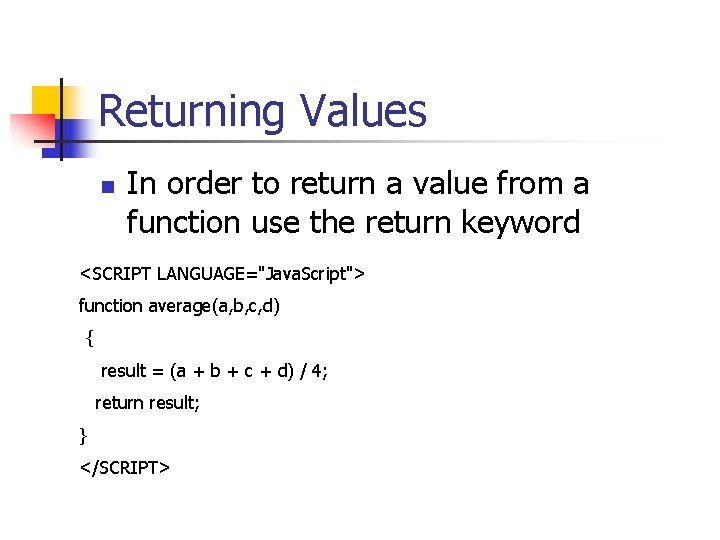Returning Values n In order to return a value from a function use the return keyword <SCRIPT LANGUAGE="Java. Script"> function average(a, b, c, d) { result = (a + b + c + d) / 4; return result; } </SCRIPT>Returning Values n To use the return value you can either n n Store the value in a variable Use the function in an expression score = average(3, 4, 5, 6); or alert(average(3, 4, 5, 6));Variables n n n Variables can include letters of the alphabet digits 0 -9 and underscore Cannot include spaces or other special characters First char must be letter of the underscore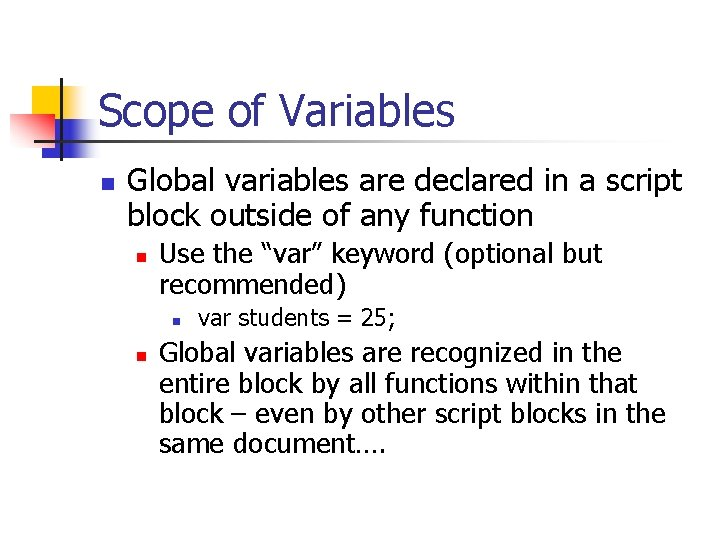Scope of Variables n Global variables are declared in a script block outside of any function n Use the “var” keyword (optional but recommended) n n var students = 25; Global variables are recognized in the entire block by all functions within that block – even by other script blocks in the same document….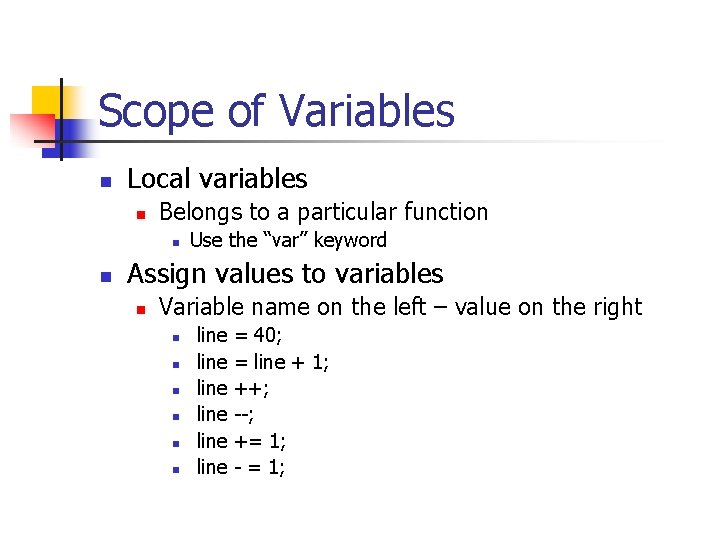Scope of Variables n Local variables n Belongs to a particular function n n Use the “var” keyword Assign values to variables n Variable name on the left – value on the right n n n line line = 40; = line + 1; ++; --; += 1; - = 1;Data Types n Java. Script will allow the following types of data to be stored in variables n n n Numbers – integers and floating point Boolean – true/false Strings – one or more characters of text Null value - keyword “null” no value Variables in web pages are not specifically “typed” n They are “variants” – will hold data of any typeData Types n You can change the type of data a variable holds by assigning it a new value n n total = 41; total = “This is a string”; n n This is legal in Java. Script When it is necessary to convert data into numbers for math use: n n parse. Int() converts a string into an integer parse. Float() converts a string to a floating-point number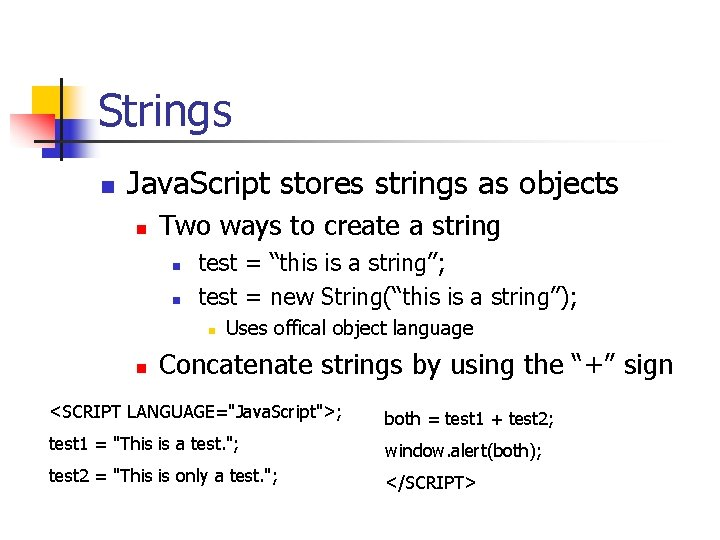Strings n Java. Script stores strings as objects n Two ways to create a string n n test = “this is a string”; test = new String(“this is a string”); n n Uses offical object language Concatenate strings by using the “+” sign <SCRIPT LANGUAGE="Java. Script">; both = test 1 + test 2; test 1 = "This is a test. "; window. alert(both); test 2 = "This is only a test. "; </SCRIPT>Calculating the String Length n Use the string name and the “length” method n n test = “this is a string”; document. write(test. length);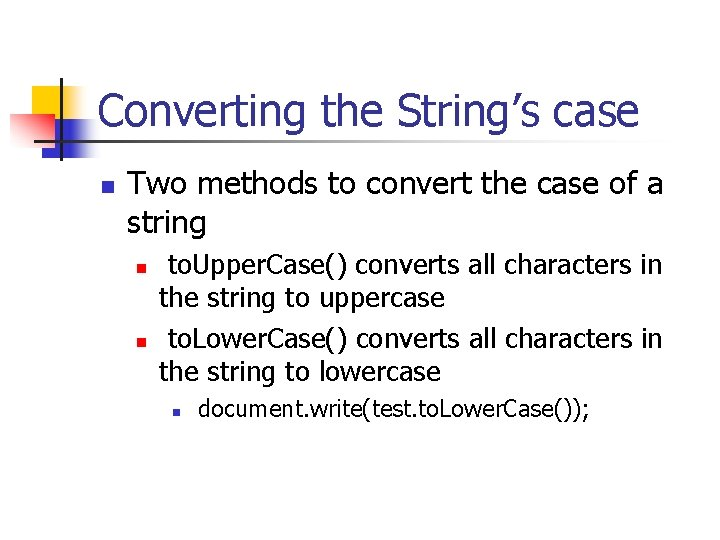Converting the String’s case n Two methods to convert the case of a string n n to. Upper. Case() converts all characters in the string to uppercase to. Lower. Case() converts all characters in the string to lowercase n document. write(test. to. Lower. Case());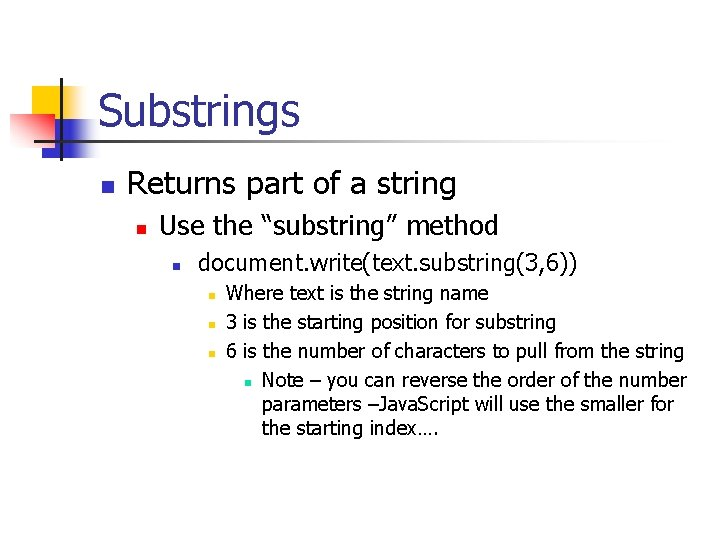Substrings n Returns part of a string n Use the “substring” method n document. write(text. substring(3, 6)) n n n Where text is the string name 3 is the starting position for substring 6 is the number of characters to pull from the string n Note – you can reverse the order of the number parameters –Java. Script will use the smaller for the starting index….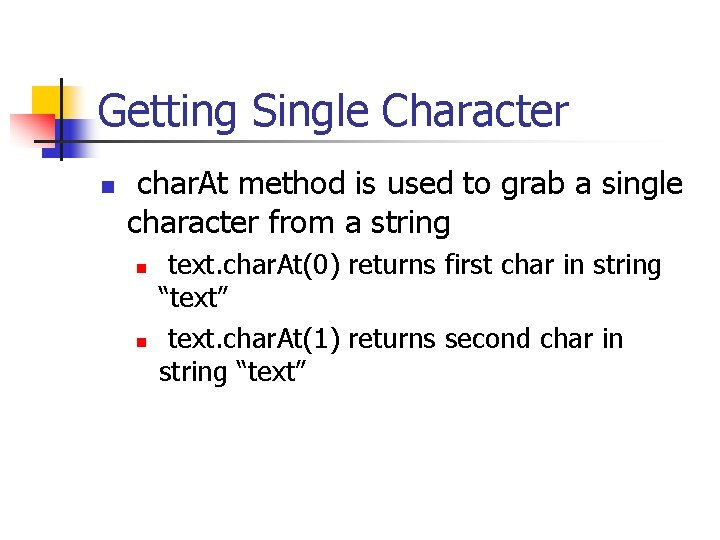Getting Single Character n char. At method is used to grab a single character from a string n n text. char. At(0) returns first char in string “text” text. char. At(1) returns second char in string “text”Finding a Substring n You can find a substring within a string by using the “index. Of” method n n n location = text. index. Of(“tst”); Will return the value of the position of first occurance of the substring “tst” in “text”. location = text. index. Of(“fish”, 19); n Will start looking for “fish” at position 20 of the string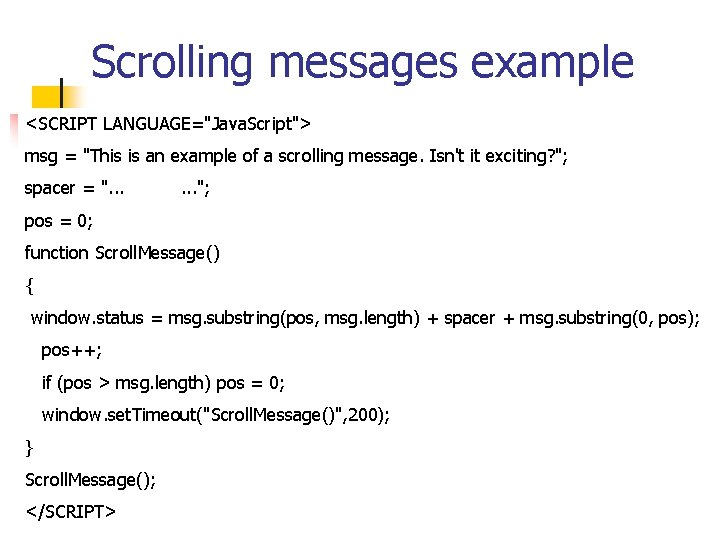Scrolling messages example <SCRIPT LANGUAGE="Java. Script"> msg = "This is an example of a scrolling message. Isn't it exciting? "; spacer = ". . . "; pos = 0; function Scroll. Message() { window. status = msg. substring(pos, msg. length) + spacer + msg. substring(0, pos); pos++; if (pos > msg. length) pos = 0; window. set. Timeout("Scroll. Message()", 200); } Scroll. Message(); </SCRIPT>Scrolling messages example </HEAD> <BODY> <H 1>Scrolling Message Example</H 1> Look at the status line at the bottom of this page. (Don't watch it too long, as it may be hypnotic. ) </BODY></HTML>Now you try: n Add a scrolling message at the bottom of a main page. n n n Use Java. Script and the previous example to do this. The message can say whatever you like The message should scroll every. 5 seconds….Branching n Decisions are coded using the “IF” syntax followed by a relational expression: if (var 1 == var 2) document. writeln(“The first value is greater than the second. ”); // Note the semicolon at the end of the branching statement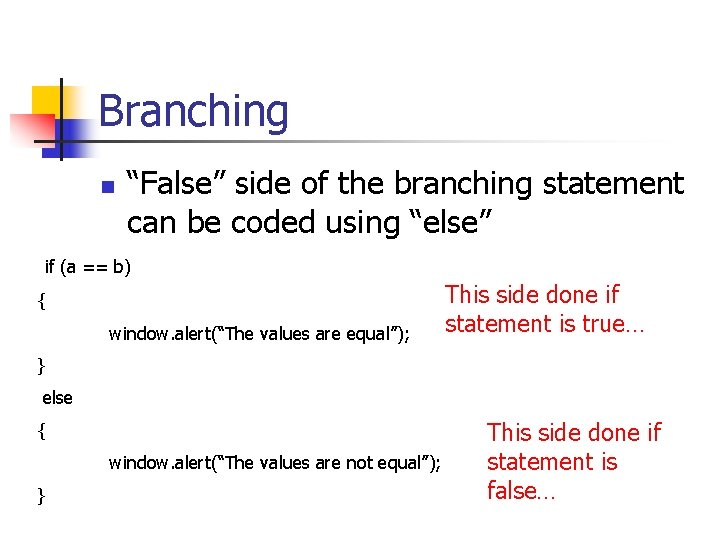Branching n “False” side of the branching statement can be coded using “else” if (a == b) { window. alert(“The values are equal”); This side done if statement is true… } else { window. alert(“The values are not equal”); } This side done if statement is false…Relational Operators n n n == Equal to != Not equal to > Greater than < Less than >= Greater than or equal to <= Less than or equal to n Used in relational expressions for branching or looping….Branching n Block statements can be created using braces and multiple statements: if (var 1 == 10) { window. alert(“Var 1 is 10!); var 1 = 0; } else { window. alert(“Invalid entry”); } Note two statements on the true side…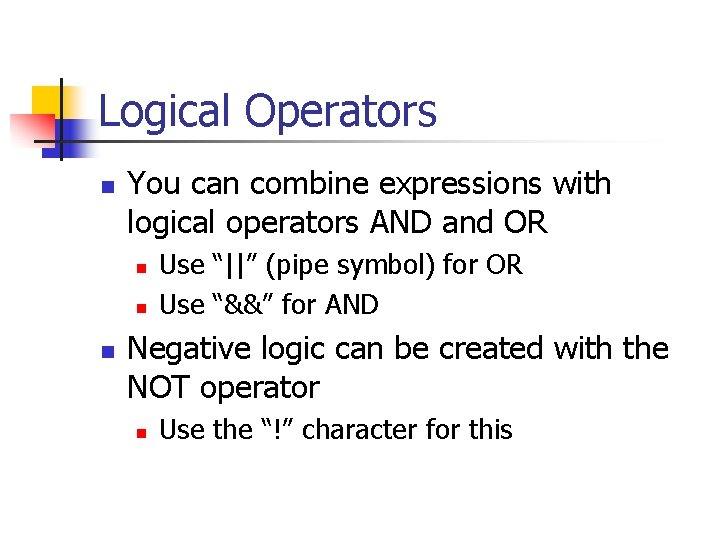Logical Operators n You can combine expressions with logical operators AND and OR n n n Use “||” (pipe symbol) for OR Use “&&” for AND Negative logic can be created with the NOT operator n Use the “!” character for this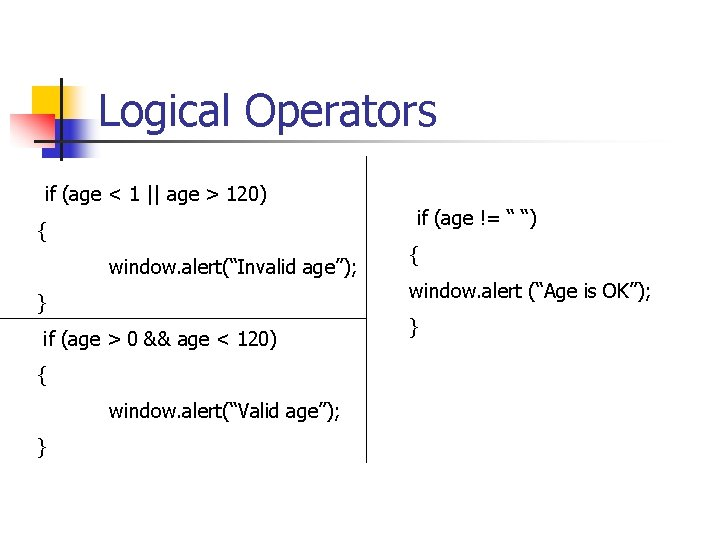Logical Operators if (age < 1 || age > 120) { window. alert(“Invalid age”); } if (age > 0 && age < 120) { window. alert(“Valid age”); } if (age != “ “) { window. alert (“Age is OK”); }Switch Statement n In order to test the same variable for a variety of different values use Switch statement n n n Initial switch statement Entire statement is enclosed in {} Uses the “case” statement “break” statement used to redirect logic Optional “default” statement for default logicSwitch Example switch(var 1) case “o”: { ; case “a”: case “u”: ; window. alert(“var 1 is a vowel”); case “e”: break; ; default: case “i”: ; window. alert(“var 1 is a consonant”); }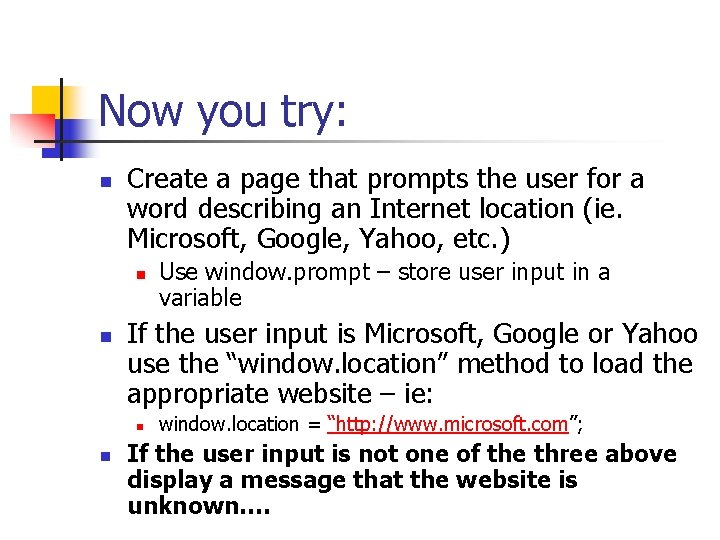Now you try: n Create a page that prompts the user for a word describing an Internet location (ie. Microsoft, Google, Yahoo, etc. ) n n If the user input is Microsoft, Google or Yahoo use the “window. location” method to load the appropriate website – ie: n n Use window. prompt – store user input in a variable window. location = “http: //www. microsoft. com”; If the user input is not one of the three above display a message that the website is unknown….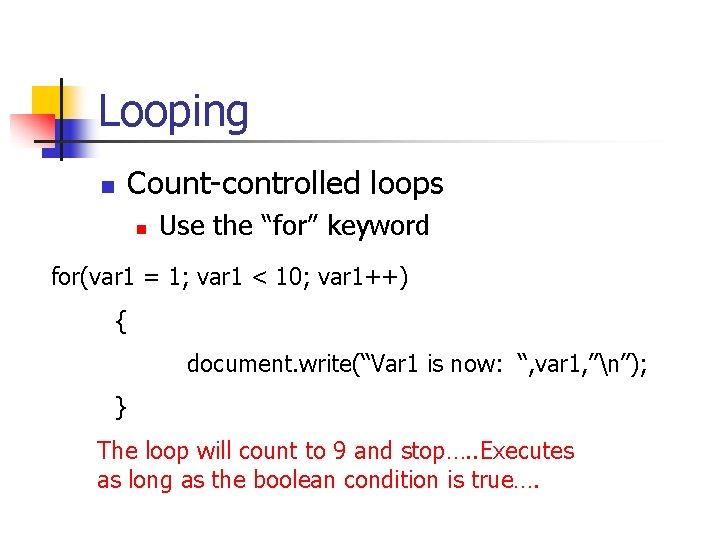Looping Count-controlled loops n n Use the “for” keyword for(var 1 = 1; var 1 < 10; var 1++) { document. write(“Var 1 is now: “, var 1, ”n”); } The loop will count to 9 and stop…. . Executes as long as the boolean condition is true….For “in” statement for (i in names) { document. write("<LI>" + names[i] + "<BR>"); } Allows looping through an array or object using the “in” operator…. Assuming “names” is an array that has been loaded….While Loop n Use keyword “while” n n n Leading decision Executes as long as the condition is true Does not use a counter while (fname != “end”) { var 1 += 1; fname= window. prompt(“Enter your name”, ”end”); }Do While Loop n Uses the keywords “do” and “while” n n n Trailing decision Loop will execute at least once Loops until the while condition is true do { next = window. prompt(“enter a name”); if (next > “ “) names[i] = next; i++; } while(next > “ “);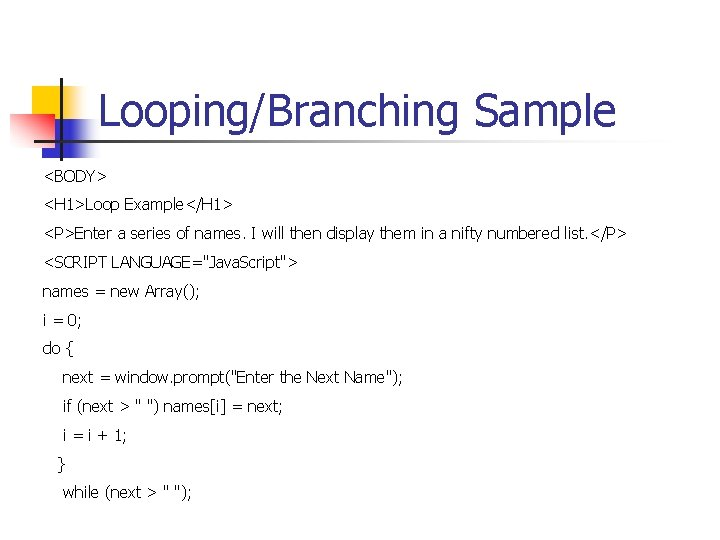Looping/Branching Sample <BODY> <H 1>Loop Example</H 1> <P>Enter a series of names. I will then display them in a nifty numbered list. </P> <SCRIPT LANGUAGE="Java. Script"> names = new Array(); i = 0; do { next = window. prompt("Enter the Next Name"); if (next > " ") names[i] = next; i = i + 1; } while (next > " ");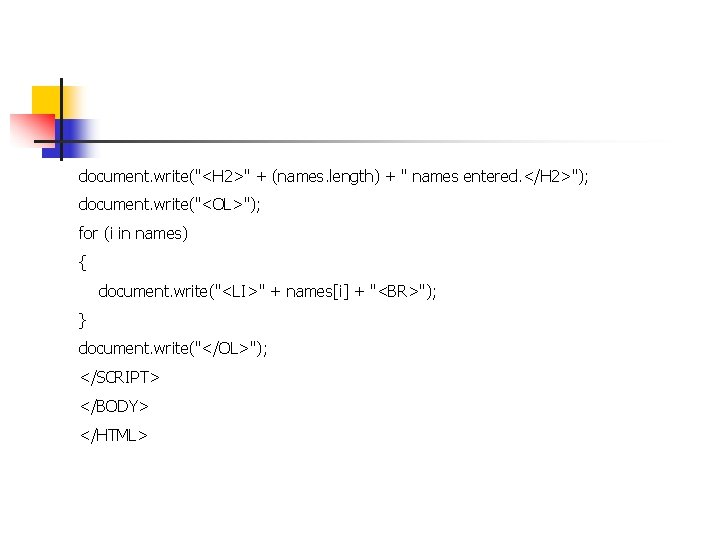document. write("<H 2>" + (names. length) + " names entered. </H 2>"); document. write("<OL>"); for (i in names) { document. write("<LI>" + names[i] + "<BR>"); } document. write("</OL>"); </SCRIPT> </BODY> </HTML>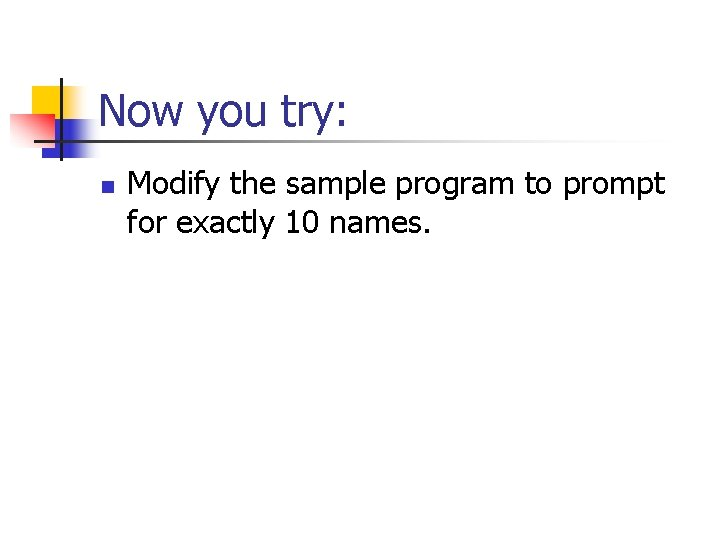Now you try: n Modify the sample program to prompt for exactly 10 names.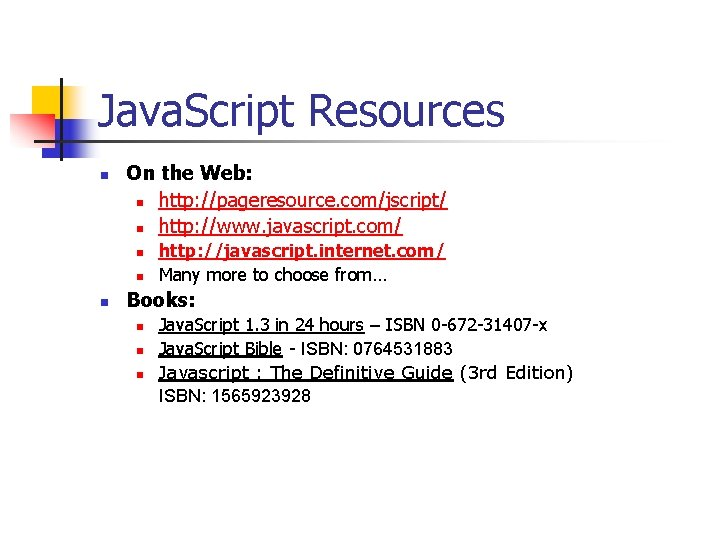Java. Script Resources n On the Web: n http: //pageresource. com/jscript/ n http: //www. javascript. com/ n n n http: //javascript. internet. com/ Many more to choose from… Books: n n n Java. Script 1. 3 in 24 hours – ISBN 0 -672 -31407 -x Java. Script Bible - ISBN: 0764531883 Javascript : The Definitive Guide (3 rd Edition) ISBN: 1565923928